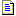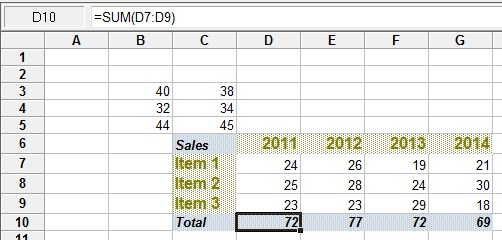﻿ Visual DAQ DaqPlaner - SpreadSheet - Formula Calculation

#SpreadSheet - Formula Calculation

Formulas are the basic building blocks for analyzing and calculating worksheet data. A formula is a string containing numbers, operators, worksheet functions, cell references, and names. A formula can contain as many as 1024 characters.

• To enter a formula in a cell, begin the entry with an equal sign (=). Then type the function name and staring parenthesis. Type the cell reference or you select the affected cells with the mouse selection. Type any other arguments, if any and complete the parenthesis. Then type enter to finish editing the cell.

• If text is encountered when a number is expected, the text is converted to a number. For example, the formula 1 + "3" returns 4, because "3" is converted to a number. If the text cannot be converted to a valid number (e.g., 1 + "Text"), #VALUE! is returned.

• Likewise, if a number is encountered when text is expected, the number is converted to text. The formula "The number is "&3 converts to the text string "The number is 3".

• To copy the formula to another cell, copy the formula from the formula bar, paste it to target cell and change the cell reference within the formula.

1. Formula Bar(Figure 1 : Formula Bar)

2. Common Formula Functions

Following are the most commonly used formula functions:

 Category Common Functions Statistical AVERAGE, SUM, STDEV, COUNT, COUNTIF, MAX, MIN Mathematical MOD, CEILING, FLOOR, EXP, FACT, LOG, LOG10, SQRT, TRUNC, ROUND Text ASC, CONCATENATE, LEFT, LEN, FIND, LOWER, UPPER, MATCH, MID, REPLACE Trigonometric COS, COSH, ACOS, ACOSH etc. Logical AND, OR, NOT Date & Time DATE, DATEVALUE, DAY, DAYS360, MONTH, YEAR, HOUR, MINUTE, TIME, TIMEVALUE, TODAY, NOW

3. Formula Operators

Arithmetic

 Operator Meaning + Addition - Subtraction / Division * Multiplication % Percentage ^ Exponentiation

Text Comparison

 Operator Meaning & Concatenation = Equal to > Greater than < Less than >= Greater than or equal to <= Less than or equal to <> Not equal to

Reference

 Operator Meaning :, .., . Range - produces a reference that includes all the cells between the two references (e.g., A1:A5 includes cells A1 and A5 and all cells in between). , Union - produces one reference that includes the two references (e.g., A1:A10,C1:C10).

4. Formula Operator Precedence

The following table lists the order of precedence for formula operators.

 Operator Description ( ) Parentheses :, .., . Range , Union - Negation (single operand) % Percentage ^ Exponentiation * and / Multiplication and Division + and - Addition and Subtraction & Text concatenation = < > <= >= <> Comparison

Operators of like precedence are evaluated left to right. Parentheses should be used when it is necessary to change the order of evaluation. The following example illustrates how the result of a formula can be altered by adding parentheses to change the order of precedence.

 Formula Result 1+2*37        75 7551 (1+2)*37 111

As illustrated in the previous table, the multiplication operator (*) has higher precedence than the addition operator (+). It is evaluated first unless parentheses are used to force the addition to take place first.